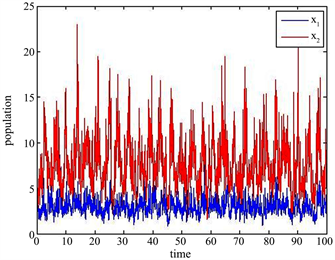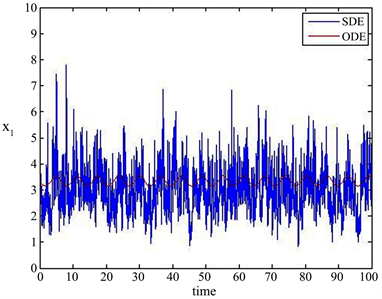# 一类非自治随机互惠系统的渐近性态Asymptotic Behavior of a Non-Autonomous Stochastic Mutualism System

DOI: 10.12677/PM.2019.94068, PDF, HTML, XML, 下载: 285  浏览: 402  国家自然科学基金支持

Abstract: This paper is devoted to the asymptotic behavior of a non-autonomous stochastic mutualism system. Firstly, the existence and uniqueness of global positive solution to the system is established for any positive initial value. Then by using the comparison theorem for stochastic differential equations and Lyapunov functions, the sufficient conditions for the permanence, extinction, global attractivity, and existence of periodic solutions to the system are derived respectively. Finally, some numerical simulations are given to illustrate our theoretical results.

1. 引言

2006年，Gravesa等人  提出以下互惠模型

$\left\{\begin{array}{l}\frac{\text{d}{x}_{1}\left(t\right)}{\text{d}t}={x}_{1}\left(t\right)\left[{r}_{1}-{b}_{1}{\text{e}}^{-{k}_{1}{x}_{2}\left(t\right)}-{c}_{1}{x}_{1}\left(t\right)\right],\hfill \\ \frac{\text{d}{x}_{2}\left(t\right)}{\text{d}t}={x}_{2}\left(t\right)\left[{r}_{2}-{b}_{2}{\text{e}}^{-{k}_{2}{x}_{1}\left(t\right)}-{c}_{2}{x}_{2}\left(t\right)\right],\hfill \end{array}$ (1.1)

$\left\{\begin{array}{l}\frac{\text{d}{x}_{1}\left(t\right)}{\text{d}t}={x}_{1}\left(t\right)\left[{r}_{1}\left(t\right)-{b}_{1}\left(t\right){\text{e}}^{-{k}_{1}\left(t\right){x}_{2}\left(t\right)}-{c}_{1}\left(t\right){x}_{1}\left(t\right)\right],\hfill \\ \frac{\text{d}{x}_{2}\left(t\right)}{\text{d}t}={x}_{2}\left(t\right)\left[{r}_{2}\left(t\right)-{b}_{2}\left(t\right){\text{e}}^{-{k}_{2}\left(t\right){x}_{1}\left(t\right)}-{c}_{2}\left(t\right){x}_{2}\left(t\right)\right],\hfill \end{array}$ (1.2)

$\left\{\begin{array}{c}\text{d}{x}_{1}\left(t\right)={x}_{1}\left(t\right)\left[{r}_{1}-{b}_{1}{\text{e}}^{-{k}_{1}{x}_{2}\left(t\right)}-{c}_{1}{x}_{1}\left(t\right)\right]\text{d}t+{\sigma }_{1}{x}_{1}\left(t\right)\text{d}{B}_{1}\left(t\right),\\ \text{d}{x}_{2}\left(t\right)={x}_{2}\left(t\right)\left[{r}_{2}-{b}_{2}{\text{e}}^{-{k}_{2}{x}_{1}\left(t\right)}-{c}_{2}{x}_{2}\left(t\right)\right]\text{d}t+{\sigma }_{2}{x}_{2}\left(t\right)\text{d}{B}_{2}\left(t\right),\end{array}$ (1.3)

$\left\{\begin{array}{l}\text{d}{x}_{1}\left(t\right)={x}_{1}\left(t\right)\left[{r}_{1}\left(t\right)-{b}_{1}\left(t\right){\text{e}}^{-{k}_{1}\left(t\right){x}_{2}\left(t\right)}-{c}_{1}\left(t\right){x}_{1}\left(t\right)\right]\text{d}t+{\sigma }_{1}\left(t\right){x}_{1}\left(t\right)\text{d}{B}_{1}\left(t\right),\\ \text{d}{x}_{2}\left(t\right)={x}_{2}\left(t\right)\left[{r}_{2}\left(t\right)-{b}_{2}\left(t\right){\text{e}}^{-{k}_{2}\left(t\right){x}_{1}\left(t\right)}-{c}_{2}\left(t\right){x}_{2}\left(t\right)\right]\text{d}t+{\sigma }_{2}\left(t\right){x}_{2}\left(t\right)\text{d}{B}_{2}\left(t\right),\end{array}$ (1.4)

1) ${R}_{+}^{2}=\left\{\left({x}_{1},{x}_{2}\right):{x}_{1}>0,{x}_{2}>0\right\}$

2) 对 $\left[0,\infty \right)$ 上的有界函数f，定义 $\stackrel{⌣}{f}=\underset{t\in \left[0,\infty \right)}{\mathrm{sup}}f\left(t\right),\stackrel{⌢}{f}=\underset{t\in \left[0,\infty \right)}{\mathrm{inf}}f\left(t\right)$

3) 给定 $\theta >0$，对 $\left[0,\theta \right]$ 上的可积函数g，定义 ${〈g〉}_{\theta }=\frac{1}{\theta }{\int }_{0}^{\theta }g\left(s\right)\text{d}s$

2. 正解的存在唯一性

${\tau }_{m}=\mathrm{inf}\left\{t\in \left[0,{\tau }_{e}\right):{x}_{1}\left(t\right)\notin \left(\frac{1}{m},m\right)或者{x}_{2}\left(t\right)\notin \left(\frac{1}{m},m\right)\right\}.$

$ℙ\left\{{\tau }_{\infty }\le T\right\}>\epsilon$ .

$ℙ\left\{{\tau }_{m}\le T\right\}\ge \epsilon$ . (2.1)

$V\left({x}_{1},{x}_{2}\right)=\left({x}_{1}-1-\mathrm{ln}{x}_{1}\right)+\left({x}_{2}-1-\mathrm{ln}{x}_{2}\right).$

$\text{d}V\left({x}_{1},{x}_{2}\right)=LV\left({x}_{1},{x}_{2}\right)+\left({x}_{1}-1\right){\sigma }_{1}\left(t\right)\text{d}{B}_{1}\left(t\right)+\left({x}_{2}-1\right){\sigma }_{2}\left(t\right)\text{d}{B}_{2}\left(t\right),$ (2.2)

$\begin{array}{c}LV\left({x}_{1},{x}_{2}\right)=\left({x}_{1}-1\right)\left[{r}_{1}\left(t\right)-{b}_{1}\left(t\right){\text{e}}^{-{k}_{1}\left(t\right){x}_{2}\left(t\right)}-{c}_{1}\left(t\right){x}_{1}\left(t\right)\right]\\ \text{\hspace{0.17em}}\text{\hspace{0.17em}}+\left({x}_{2}-1\right)\left[{r}_{2}\left(t\right)-{b}_{2}\left(t\right){\text{e}}^{-{k}_{2}\left(t\right){x}_{1}\left(t\right)}-{c}_{2}\left(t\right){x}_{2}\left(t\right)\right]+\text{0}\text{.5}\left({\sigma }_{1}^{2}\left(t\right)+{\sigma }_{2}^{2}\left(t\right)\right)\\ \le {x}_{1}\left({\stackrel{⌣}{r}}_{1}+{\stackrel{⌣}{c}}_{1}-{\stackrel{⌢}{c}}_{1}{x}_{1}\right)+{x}_{2}\left({\stackrel{⌣}{r}}_{2}+{\stackrel{⌣}{c}}_{2}-{\stackrel{⌢}{c}}_{2}{x}_{2}\right)+{\stackrel{⌣}{b}}_{1}+{\stackrel{⌣}{b}}_{2}+0.5\left({\stackrel{⌣}{\sigma }}_{1}^{2}+{\stackrel{⌣}{\sigma }}_{2}^{2}\right)\\ =:G\left({x}_{1},{x}_{2}\right).\end{array}$ (2.3)

$\mathbb{E}V\left({x}_{1}\left({\tau }_{m}\wedge T\right),{x}_{2}\left({\tau }_{m}\wedge T\right)\right)=V\left({x}_{1}\left(0\right),{x}_{2}\left(0\right)\right)+\mathbb{E}{\int }_{0}^{{\tau }_{m}\wedge T}LV\text{d}t\le V\left({x}_{1}\left(0\right),{x}_{2}\left(0\right)\right)+MT.$ (2.4)

$m\ge {m}_{1}$，记 ${\Omega }_{m}=\left\{{\tau }_{m}\le T\right\}$。由 ${\tau }_{m}$ 的定义可知，对每个 $\omega \in {\Omega }_{m}$，存在 $x\left({\tau }_{m},\omega \right)$ 的某个分量 ${x}_{i}\left({\tau }_{m},\omega \right)\left(i=1或2\right)$ 等于m或者1/m。结合(2.1)，有

$\mathbb{E}V\left({x}_{1}\left({\tau }_{m}\wedge T\right),{x}_{2}\left({\tau }_{m}\wedge T\right)\right)\ge \mathbb{E}\left[{I}_{{\Omega }_{m}}V\left({x}_{1}\left({\tau }_{m}\wedge T\right),{x}_{2}\left({\tau }_{m}\wedge T\right)\right)\right]\ge \epsilon \left[m-1-\mathrm{ln}m\right]\wedge \left[\mathrm{ln}m-1+\frac{1}{m}\right],$ (2.5)

$V\left({x}_{1}\left(0\right),{x}_{2}\left(0\right)\right)+MT\ge \epsilon \left[m-1-\mathrm{ln}m\right]\wedge \left[\mathrm{ln}m-1+\frac{1}{m}\right].$

$m\to \infty$，有

$V\left({x}_{1}\left(0\right),{x}_{2}\left(0\right)\right)+MT\ge \infty ,$

3. 全局吸引性

$\mathbb{E}{|x\left(t\right)-x\left(s\right)|}^{\alpha }\le c{|t-s|}^{1+\beta },\text{\hspace{0.17em}}\text{\hspace{0.17em}}0\le s,t<+\infty ,$

$P\left\{\omega :\underset{0<|t-s|

$\begin{array}{l}\text{d}\left({\text{e}}^{t}{x}_{1}^{p}\right)={\text{e}}^{t}{x}_{1}^{p}\left[1+p\left({r}_{1}\left(t\right)-{b}_{1}\left(t\right){\text{e}}^{-{k}_{1}\left(t\right){x}_{2}}-{c}_{1}\left(t\right){x}_{1}\right)+0.5p\left(p-1\right){\sigma }_{1}^{2}\left(t\right)\right]\text{d}t+p{\text{e}}^{t}{x}_{1}^{p}{\sigma }_{1}\text{d}{B}_{1}\left(t\right)\\ \text{}\le {\text{e}}^{t}{x}_{1}^{p}\left[1+p{\stackrel{⌣}{r}}_{1}+0.5p\left(p-1\right){\stackrel{⌣}{\sigma }}_{1}^{2}-p{\stackrel{⌢}{c}}_{1}{x}_{1}\right]\text{d}t+p{\text{e}}^{t}{x}_{1}^{p}{\sigma }_{1}\left(t\right)\text{d}{B}_{1}\left(t\right)\end{array}$ (3.1)

$H\left(x\right)={x}^{p}\left[1+p{\stackrel{⌣}{r}}_{1}+0.5p\left(p-1\right){\stackrel{⌣}{\sigma }}_{1}^{2}-p{\stackrel{⌢}{c}}_{1}x\right]$。易知 $H\left(x\right)$ 在上 $\left(0,\infty \right)$ 有正上界，记为 $K\left(p\right)$。对(3.1)两边从0到t积分，并取数学期望得

${\text{e}}^{t}\mathbb{E}{x}_{1}^{p}\left(t\right)-\mathbb{E}{x}_{1}^{p}\left(0\right)\le {\int }_{0}^{t}{\text{e}}^{s}H\left({x}_{1}\left(s\right)\right)\text{d}s\le K\left(p\right){\text{e}}^{t}.$

$\underset{t\to \infty }{\mathrm{lim}\mathrm{sup}}\mathbb{E}{x}_{1}^{p}\left(t\right)\le K\left(p\right).$

$\mathbb{E}{x}_{1}^{p}\left(t\right)\le \stackrel{˜}{K}\left(p,{x}_{1}\left(0\right)\right).$ (3.2)

${x}_{1}\left(s\right)={x}_{1}\left(0\right)+{\int }_{0}^{t}{f}_{1}\left(s\right)\text{d}s+{\int }_{0}^{t}{g}_{1}\left(s\right)\text{d}{B}_{1}\left(s\right),$ (3.3)

${f}_{1}\left(s\right)={x}_{1}\left(s\right)\left[{r}_{1}\left(s\right)-{b}_{1}\left(s\right){\text{e}}^{-{k}_{1}\left(s\right){x}_{2}\left(s\right)}-{c}_{1}\left(s\right){x}_{1}\left(s\right)\right],\text{\hspace{0.17em}}{g}_{1}\left(s\right)={\sigma }_{1}\left(s\right){x}_{1}\left(s\right).$

$\begin{array}{c}\mathbb{E}{|{f}_{1}\left(s\right)|}^{p}=\mathbb{E}\left({x}_{1}^{p}\left(s\right){|{r}_{1}\left(s\right)-{b}_{1}\left(s\right){\text{e}}^{-{k}_{1}\left(s\right){x}_{2}\left(s\right)}-{c}_{1}\left(s\right){x}_{1}\left(s\right)|}^{p}\right)\\ \le 0.5\mathbb{E}{x}_{1}^{2p}\left(s\right)+0.5\mathbb{E}{|{r}_{1}\left(s\right)-{b}_{1}\left(s\right){\text{e}}^{-{k}_{1}\left(s\right){x}_{2}\left(s\right)}-{c}_{1}\left(s\right){x}_{1}\left(s\right)|}^{2p}\\ \le 0.5\mathbb{E}{x}_{1}^{2p}\left(s\right)+{2}^{2p-2}{\left({\stackrel{⌣}{r}}_{1}+{\stackrel{⌣}{b}}_{1}\right)}^{2p}+{2}^{2p-2}{\stackrel{⌣}{c}}_{1}\mathbb{E}{x}_{1}^{2p}\left(s\right)\\ \le \left(0.5+{2}^{2p-2}{\stackrel{⌣}{c}}_{1}\right)\stackrel{˜}{K}\left(2p,{x}_{1}\left(0\right)\right)+{2}^{2p-2}{\left({\stackrel{⌣}{r}}_{1}+{\stackrel{⌣}{b}}_{1}\right)}^{2p},\end{array}$ (3.4)

$\mathbb{E}{|{g}_{1}\left(s\right)|}^{p}=\mathbb{E}\left({\sigma }_{1}^{p}\left(s\right){x}_{1}^{p}\left(s\right)\right)\le {\stackrel{⌣}{\sigma }}_{1}{}^{p}\mathbb{E}{x}_{1}^{p}\left(s\right)\le {\stackrel{⌣}{\sigma }}_{1}^{p}\stackrel{˜}{K}\left(p,{x}_{1}\left(0\right)\right).$ (3.5)

$\begin{array}{c}\mathbb{E}{|{\int }_{{t}_{1}}^{{t}_{2}}{g}_{1}\left(s\right)\text{d}{B}_{1}\left(s\right)|}^{p}\le {\left[\frac{p\left(p-1\right)}{2}\right]}^{\frac{p}{2}}{\left({t}_{2}-{t}_{1}\right)}^{\frac{p-2}{2}}{\int }_{{t}_{1}}^{{t}_{2}}\mathbb{E}{|{g}_{1}\left(s\right)|}^{p}\text{d}s\\ \le {\stackrel{⌣}{\sigma }}_{1}^{p}\stackrel{˜}{K}\left(p,{x}_{1}\left(0\right)\right){\left[\frac{p\left(p-1\right)}{2}\right]}^{\frac{p}{2}}{\left({t}_{2}-{t}_{1}\right)}^{\frac{p}{2}}.\end{array}$ (3.6)

$\frac{1}{p}+\frac{1}{q}=1,$

$\begin{array}{c}\mathbb{E}{\left({\int }_{{t}_{1}}^{{t}_{2}}|{f}_{1}\left(s\right)|\text{d}s\right)}^{p}\le \mathbb{E}{\left\{{\left({\int }_{{t}_{1}}^{{t}_{2}}{1}^{q}\text{d}s\right)}^{\frac{1}{q}}\cdot {\left({\int }_{{t}_{1}}^{{t}_{2}}{|{f}_{1}\left(s\right)|}^{p}\text{d}s\right)}^{\frac{1}{p}}\right\}}^{p}={\left({t}_{2}-{t}_{1}\right)}^{\frac{p}{q}}\cdot {\int }_{{t}_{1}}^{{t}_{2}}\mathbb{E}{|{f}_{1}\left(s\right)|}^{p}\text{d}s\\ \le \left[\left(0.5+{2}^{2p-2}{\stackrel{⌣}{c}}_{1}\right)\stackrel{˜}{K}\left(2p,{x}_{1}\left(0\right)\right)+{2}^{2p-2}{\left({\stackrel{⌣}{r}}_{1}+{\stackrel{⌣}{b}}_{1}\right)}^{2p}\right]{\left({t}_{2}-{t}_{1}\right)}^{p}.\end{array}$ (3.7)

$\begin{array}{l}\mathbb{E}{|{x}_{1}\left({t}_{2}\right)-{x}_{1}\left({t}_{1}\right)|}^{p}\\ \le {2}^{p-1}\mathbb{E}{\left({\int }_{{t}_{1}}^{{t}_{2}}|{f}_{1}\left(s\right)|\text{d}s\right)}^{p}+{2}^{p-1}\mathbb{E}{|{\int }_{{t}_{1}}^{{t}_{2}}{g}_{1}\left(s\right)\text{d}{B}_{1}\left(s\right)|}^{p}\\ \le {2}^{p-1}\left\{\left(0.5+{2}^{2p-2}{\stackrel{⌣}{c}}_{1}\right)\stackrel{˜}{K}\left(2p,{x}_{1}\left(0\right)\right)+{2}^{2p-2}{\left({\stackrel{⌣}{r}}_{1}+{\stackrel{⌣}{b}}_{1}\right)}^{2p}+{\stackrel{⌣}{\sigma }}_{1}^{p}\stackrel{˜}{K}\left(p,{x}_{1}\left(0\right)\right){\left[\frac{p\left(p-1\right)}{2}\right]}^{\frac{p}{2}}\right\}{\left({t}_{2}-{t}_{1}\right)}^{\frac{p}{2}}.\end{array}$

$\mu =\mathrm{min}\left\{\underset{t\ge 0}{\mathrm{inf}}\left[{c}_{1}\left(t\right)-{b}_{2}\left(t\right){k}_{2}\left(t\right)\right],\underset{t\ge 0}{\mathrm{inf}}\left[{c}_{2}\left(t\right)-{b}_{1}\left(t\right){k}_{1}\left(t\right)\right]\right\},$

$\mu >0$。设 $x\left(t\right)=\left({x}_{1}\left(t\right),{x}_{2}\left(t\right)\right)$$\stackrel{¯}{x}\left(t\right)=\left({\stackrel{¯}{x}}_{1}\left(t\right),{\stackrel{¯}{x}}_{2}\left(t\right)\right)$ 分别系统(1.4)的初值为 $x\left(0\right)$$\stackrel{¯}{x}\left(0\right)$ 的两个解，由Itô公式和Lagrange中值定理，可得

$\begin{array}{c}d\left(\mathrm{ln}{x}_{1}\left(t\right)-\mathrm{ln}{\stackrel{¯}{x}}_{1}\left(t\right)\right)=\left\{-{b}_{1}\left(t\right)\left[{\text{e}}^{-{k}_{1}\left(t\right){x}_{2}\left(t\right)}-{\text{e}}^{-{k}_{1}\left(t\right){\stackrel{¯}{x}}_{2}\left(t\right)}\right]-{c}_{1}\left(t\right)\left[{x}_{1}\left(t\right)-{\stackrel{¯}{x}}_{1}\left(t\right)\right]\right\}\text{d}t\\ =\left\{{b}_{1}\left(t\right){k}_{1}\left(t\right){\text{e}}^{-{k}_{1}\left(t\right){\xi }_{1}\left(t\right)}\left[{x}_{2}\left(t\right)-{\stackrel{¯}{x}}_{2}\left(t\right)\right]-{c}_{1}\left(t\right)\left[{x}_{1}\left(t\right)-{\stackrel{¯}{x}}_{1}\left(t\right)\right]\right\}\text{d}t,\end{array}$ (3.8)

$\begin{array}{c}d\left(\mathrm{ln}{x}_{2}\left(t\right)-\mathrm{ln}{\stackrel{¯}{x}}_{2}\left(t\right)\right)=\left\{-{b}_{2}\left(t\right)\left[{\text{e}}^{-{k}_{2}\left(t\right){x}_{1}\left(t\right)}-{\text{e}}^{-{k}_{2}\left(t\right){\stackrel{¯}{x}}_{1}\left(t\right)}\right]-{c}_{2}\left(t\right)\left[{x}_{2}\left(t\right)-{\stackrel{¯}{x}}_{2}\left(t\right)\right]\right\}\text{d}t\\ =\left\{{b}_{2}\left(t\right){k}_{2}\left(t\right){\text{e}}^{-{k}_{2}\left(t\right){\xi }_{2}\left(t\right)}\left[{x}_{1}\left(t\right)-{\stackrel{¯}{x}}_{1}\left(t\right)\right]-{c}_{2}\left(t\right)\left[{x}_{2}\left(t\right)-{\stackrel{¯}{x}}_{2}\left(t\right)\right]\right\}\text{d}t,\end{array}$ (3.9)

${V}_{2}\left(t\right)=|\mathrm{ln}{x}_{1}\left(t\right)-\mathrm{ln}{\stackrel{¯}{x}}_{1}\left(t\right)|+|\mathrm{ln}{x}_{2}\left(t\right)-\mathrm{ln}{\stackrel{¯}{x}}_{2}\left(t\right)|,$

$\begin{array}{l}{V}_{2}\left(t\right)-{V}_{2}\left(0\right)\\ ={\int }_{0}^{t}\mathrm{sgn}\left(\mathrm{ln}{x}_{1}\left(s\right)-\mathrm{ln}{\stackrel{¯}{x}}_{1}\left(s\right)\right)\text{d}\left(\mathrm{ln}{x}_{1}\left(s\right)-\mathrm{ln}{\stackrel{¯}{x}}_{1}\left(s\right)\right)\\ \text{\hspace{0.17em}}\text{\hspace{0.17em}}\text{ }+{\int }_{0}^{t}\mathrm{sgn}\left(\mathrm{ln}{x}_{2}\left(s\right)-\mathrm{ln}{\stackrel{¯}{x}}_{2}\left(s\right)\right)\text{d}\left(\mathrm{ln}{x}_{2}\left(s\right)-\mathrm{ln}{\stackrel{¯}{x}}_{2}\left(s\right)\right)\\ ={\int }_{0}^{t}\mathrm{sgn}\left({x}_{1}\left(s\right)-{\stackrel{¯}{x}}_{1}\left(s\right)\right)\left\{{b}_{1}\left(s\right){k}_{1}\left(s\right){\text{e}}^{-{k}_{1}\left(s\right){\xi }_{1}\left(s\right)}\left[{x}_{2}\left(s\right)-{\stackrel{¯}{x}}_{2}\left(s\right)\right]-{c}_{1}\left(s\right)\left[{x}_{1}\left(s\right)-{\stackrel{¯}{x}}_{1}\left(s\right)\right]\right\}\text{d}s\\ \text{\hspace{0.17em}}\text{\hspace{0.17em}}\text{ }+{\int }_{0}^{t}\mathrm{sgn}\left({x}_{2}\left(s\right)-{\stackrel{¯}{x}}_{2}\left(s\right)\right)\left\{{b}_{2}\left(s\right){k}_{2}\left(s\right){\text{e}}^{-{k}_{2}\left(s\right){\xi }_{2}\left(s\right)}\left[{x}_{1}\left(s\right)-{\stackrel{¯}{x}}_{1}\left(s\right)\right]-{c}_{2}\left(s\right)\left[{x}_{2}\left(s\right)-{\stackrel{¯}{x}}_{2}\left(s\right)\right]\right\}\text{d}s\end{array}$

$\begin{array}{l}\le {\int }_{0}^{t}\left[{b}_{1}\left(s\right){k}_{1}\left(s\right)|{x}_{2}\left(s\right)-{\stackrel{¯}{x}}_{2}\left(s\right)|-{c}_{1}\left(s\right)|{x}_{1}\left(s\right)-{\stackrel{¯}{x}}_{1}\left(s\right)|\right]\text{d}s\\ \text{\hspace{0.17em}}\text{\hspace{0.17em}}\text{ }+{\int }_{0}^{t}\left[{b}_{2}\left(s\right){k}_{2}\left(s\right)|{x}_{1}\left(s\right)-{\stackrel{¯}{x}}_{1}\left(s\right)|-{c}_{2}\left(s\right)|{x}_{2}\left(s\right)-{\stackrel{¯}{x}}_{2}\left(s\right)|\right]\text{d}s\\ \le -{\int }_{0}^{t}\left\{\left[{c}_{1}\left(s\right)-{b}_{2}\left(s\right){k}_{2}\left(s\right)\right]|{x}_{1}\left(s\right)-{\stackrel{¯}{x}}_{1}\left(s\right)|+\left[{c}_{2}\left(s\right)-{b}_{1}\left(s\right){k}_{1}\left(s\right)\right]|{x}_{2}\left(t\right)-{\stackrel{¯}{x}}_{2}\left(t\right)|\right\}\text{d}s\\ \le -\mu {\int }_{0}^{t}\left[|{x}_{1}\left(s\right)-{\stackrel{¯}{x}}_{1}\left(s\right)|+|{x}_{2}\left(s\right)-{\stackrel{¯}{x}}_{2}\left(s\right)|\right]\text{d}s.\end{array}$

${V}_{2}\left(t\right)+\mu {\int }_{0}^{t}\left[|{x}_{1}\left(s\right)-{\stackrel{¯}{x}}_{1}\left(s\right)|+|{x}_{2}\left(s\right)-{\stackrel{¯}{x}}_{2}\left(s\right)|\right]\text{d}t\le {V}_{2}\left(0\right).$

$\underset{t\to \infty }{\mathrm{lim}}\left[|{x}_{1}\left(t\right)-{\stackrel{¯}{x}}_{1}\left(t\right)|+|{x}_{2}\left(t\right)-{\stackrel{¯}{x}}_{2}\left(t\right)|\right]=0.$

4. 持续性与灭绝性

$\text{d}u\left(t\right)=u\left(t\right)\left[r\left(t\right)-a\left(t\right)u\left(t\right)\right]\text{d}t+\sigma \left(t\right)u\left(t\right)\text{d}B\left(t\right),$ (4.1)

$\underset{t\to \infty }{\mathrm{lim}\mathrm{inf}}ℙ\left\{u\left(t\right)\le \alpha \right\}\ge 1-\epsilon ,\text{}\underset{t\to \infty }{\mathrm{lim}\mathrm{inf}}ℙ\left\{u\left(t\right)\ge \beta \right\}\ge 1-\epsilon .$

$\underset{t\to \infty }{\mathrm{lim}\mathrm{inf}}\frac{1}{t}{\int }_{0}^{t}u\left(s\right)\text{d}s>0.$

$\underset{t\to \infty }{\mathrm{lim}\mathrm{inf}}P\left\{{x}_{i}\left(t\right)\le \alpha \right\}\ge 1-\epsilon ,\text{}\underset{t\to \infty }{\mathrm{lim}\mathrm{inf}}P\left\{{x}_{i}\left(t\right)\ge \beta \right\}\ge 1-\epsilon ,\text{}i=1,2.$

$\begin{array}{c}\text{d}{x}_{1}\left(t\right)={x}_{1}\left(t\right)\left[{r}_{1}\left(t\right)-{b}_{1}\left(t\right){\text{e}}^{-{k}_{1}\left(t\right){x}_{2}\left(t\right)}-{c}_{1}\left(t\right){x}_{1}\left(t\right)\right]\text{d}t+{\sigma }_{1}\left(t\right){x}_{1}\left(t\right)\text{d}{B}_{1}\left(t\right)\\ \le {x}_{1}\left(t\right)\left[{r}_{1}\left(t\right)-{c}_{1}\left(t\right){x}_{1}\left(t\right)\right]\text{d}t+{\sigma }_{1}\left(t\right){x}_{1}\left(t\right)\text{d}{B}_{1}\left(t\right),\end{array}$ (4.2)

$\begin{array}{c}\text{d}{x}_{1}\left(t\right)={x}_{1}\left(t\right)\left[{r}_{1}\left(t\right)-{b}_{1}\left(t\right){\text{e}}^{-{k}_{1}\left(t\right){x}_{2}\left(t\right)}-{c}_{1}\left(t\right){x}_{1}\left(t\right)\right]\text{d}t+{\sigma }_{1}\left(t\right){x}_{1}\left(t\right)\text{d}{B}_{1}\left(t\right)\\ \ge {x}_{1}\left(t\right)\left[{r}_{1}\left(t\right)-{b}_{1}\left(t\right)-{c}_{1}\left(t\right){x}_{1}\left(t\right)\right]\text{d}t+{\sigma }_{1}\left(t\right){x}_{1}\left(t\right)\text{d}{B}_{1}\left(t\right).\end{array}$ (4.3)

$\text{d}\Lambda \left(t\right)=\Lambda \left(t\right)\left[{r}_{1}\left(t\right)-{c}_{1}\left(t\right)\Lambda \left(t\right)\right]\text{d}t+{\sigma }_{1}\left(t\right)\Lambda \left(t\right)\text{d}{B}_{1}\left(t\right),$ (4.4)

$\text{d}\lambda \left(t\right)=\lambda \left(t\right)\left[{r}_{1}\left(t\right)-{b}_{1}\left(t\right)-{c}_{1}\left(t\right)\lambda \left(t\right)\right]\text{d}t+{\sigma }_{1}\left(t\right)\lambda \left(t\right)\text{d}{B}_{1}\left(t\right).$ (4.5)

$\Lambda \left(t\right)$$\lambda \left(t\right)$ 分别是方程(4.4)和(4.5)的初值为 $\Lambda \left(0\right)={x}_{1}\left(0\right)$$\lambda \left(0\right)={x}_{1}\left(0\right)$ 的解。根据随机微分方程的比较定理  ，由(4.2)和(4.3)得

$\lambda \left(t\right)\le {x}_{1}\left(t\right)\le \Lambda \left(t\right),\text{\hspace{0.17em}}\text{a}\text{.s}\text{.}$ (4.6)

$\underset{t\to \infty }{\mathrm{lim}\mathrm{inf}}P\left\{\Lambda \left(t\right)\le {\alpha }_{1}\right\}\ge 1-\epsilon$$\underset{t\to \infty }{\mathrm{lim}\mathrm{inf}}P\left\{\lambda \left(t\right)\ge {\beta }_{1}\right\}\ge 1-\epsilon$ (4.7)

$\underset{t\to \infty }{\mathrm{lim}\mathrm{inf}}P\left\{{x}_{1}\left(t\right)\le {\alpha }_{1}\right\}\ge 1-\epsilon$$\underset{t\to \infty }{\mathrm{lim}\mathrm{inf}}P\left\{{x}_{1}\left(t\right)\ge {\beta }_{1}\right\}\ge 1-\epsilon$ (4.8)

$\underset{t\to \infty }{\mathrm{lim}\mathrm{inf}}P\left\{{x}_{2}\left(t\right)\le {\alpha }_{2}\right\}\ge 1-\epsilon$$\underset{t\to \infty }{\mathrm{lim}\mathrm{inf}}P\left\{{x}_{2}\left(t\right)\ge {\beta }_{2}\right\}\ge 1-\epsilon$ (4.9)

$\alpha =\mathrm{max}\left\{{\alpha }_{1},{\alpha }_{2}\right\}$$\beta =\mathrm{min}\left\{{\beta }_{1},{\beta }_{2}\right\}$，由(4.8)和(4.9)可知，对任意 $i=1,2$，有

$\underset{t\to \infty }{\mathrm{lim}\mathrm{inf}}P\left\{{x}_{i}\left(t\right)\le \alpha \right\}\ge 1-\epsilon ,\text{\hspace{0.17em}}\underset{t\to \infty }{\mathrm{lim}\mathrm{inf}}P\left\{{x}_{i}\left(t\right)\ge \beta \right\}\ge 1-\epsilon .$

$\underset{t\to \infty }{\mathrm{lim}\mathrm{inf}}\frac{1}{t}{\int }_{0}^{t}{x}_{i}\left(t\right)\text{d}t>0,\text{a}\text{.s}\text{.,}\text{\hspace{0.17em}}i=1,2.$

$\underset{t\to \infty }{\mathrm{lim}\mathrm{inf}}\frac{1}{t}{\int }_{0}^{t}\left[{r}_{1}\left(s\right)-{b}_{1}\left(s\right)-0.5{\sigma }_{1}^{2}\left(s\right)\right]\text{d}s>0$

$\underset{t\to \infty }{\mathrm{lim}\mathrm{inf}}\frac{1}{t}{\int }_{0}^{t}\lambda \left(t\right)\text{d}t>0.$ (4.10)

$\underset{t\to \infty }{\mathrm{lim}\mathrm{inf}}\frac{1}{t}{\int }_{0}^{t}{x}_{1}\left(s\right)\text{d}s\ge \underset{t\to \infty }{\mathrm{lim}\mathrm{inf}}\frac{1}{t}{\int }_{0}^{t}\lambda \left(t\right)\text{d}t>0.$

$\underset{t\to \infty }{\mathrm{lim}\mathrm{inf}}\frac{1}{t}{\int }_{0}^{t}{x}_{2}\left(s\right)\text{d}s>0.$

$\underset{t\to \infty }{\mathrm{lim}\mathrm{sup}}\frac{\mathrm{ln}{x}_{i}\left(t\right)}{t}<0,\text{}i=1,2.$

$\text{d}\mathrm{ln}{x}_{1}\left(t\right)=\left[{r}_{1}\left(t\right)-{b}_{1}\left(t\right){\text{e}}^{-{k}_{1}\left(t\right){x}_{2}}-{c}_{1}\left(t\right){x}_{1}-0.5{\sigma }_{1}^{2}\left(t\right)\right]\text{d}t+{\sigma }_{1}\left(t\right)\text{d}{B}_{1}\left(t\right).$ (4.11)

$\mathrm{ln}{x}_{1}\left(t\right)\le \mathrm{ln}{x}_{1}\left(0\right)+{\int }_{0}^{t}\left[{r}_{1}\left(s\right)-0.5{\sigma }_{1}^{2}\left(s\right)\right]\text{d}s+M\left(t\right),$ (4.12)

${〈M,M〉}_{t}={\int }_{0}^{t}{\sigma }_{1}^{2}\left(s\right)\text{d}s\le {\stackrel{⌣}{\sigma }}_{1}^{2}t.$

$\underset{t\to \infty }{\mathrm{lim}}\frac{M\left(t\right)}{t}=0,\text{\hspace{0.17em}}\text{a}\text{.s}.$ (4.13)

$\underset{t\to \infty }{\mathrm{lim}\mathrm{sup}}\frac{\mathrm{ln}{x}_{1}\left(t\right)}{t}\le \underset{t\to \infty }{\mathrm{lim}\mathrm{sup}}\frac{1}{t}{\int }_{0}^{t}\left[{r}_{1}\left(s\right)-\frac{1}{2}{\sigma }_{1}^{2}\left(s\right)\right]\text{d}s<0,\text{\hspace{0.17em}}\text{a}\text{.s}.$

5. 周期解

$\text{d}x\left(t\right)=f\left(t,x\left(t\right)\right)\text{d}t+g\left(t,x\left(t\right)\right)\text{d}{B}_{t},$ (5.1)

$LV\left(t,x\right)={V}_{t}\left(t,x\right)+{V}_{x}\left(t,x\right)f\left(t,x\right)+0.5\text{trace}\left[{g}^{\text{T}}\left(t,x\right){V}_{xx}\left(t,x\right)g\left(t,x\right)\right].$

1) $\underset{k\to \infty }{\mathrm{lim}}\underset{|x|>k}{\mathrm{inf}}V\left(t,x\right)\to \infty$

2) $\underset{k\to \infty }{\mathrm{lim}}\underset{|x|>k}{\mathrm{sup}}LV\left(t,x\right)=-\infty$

${u}_{1}\left(t\right)=\mathrm{ln}{x}_{1}\left(t\right),\text{\hspace{0.17em}}\text{\hspace{0.17em}}{u}_{2}\left(t\right)=\mathrm{ln}{x}_{2}\left(t\right).$

$\left\{\begin{array}{l}\text{d}{u}_{1}\left(t\right)=\left[{r}_{1}\left(t\right)-0.5{\sigma }_{1}^{2}\left(t\right)-{b}_{1}\left(t\right){\text{e}}^{-{k}_{1}\left(t\right){\text{e}}^{{u}_{2}\left(t\right)}}-{c}_{1}\left(t\right){\text{e}}^{{u}_{1}\left(t\right)}\right]\text{d}t+{\sigma }_{1}\left(t\right)\text{d}{B}_{1}\left(t\right),\\ \text{d}{u}_{2}\left(t\right)=\left[{r}_{2}\left(t\right)-0.5{\sigma }_{2}^{2}\left(t\right)-{b}_{2}\left(t\right){\text{e}}^{-{k}_{2}\left(t\right){\text{e}}^{{u}_{1}\left(t\right)}}-{c}_{2}\left(t\right){\text{e}}^{{u}_{2}\left(t\right)}\right]\text{d}t+{\sigma }_{2}\left(t\right)\text{d}{B}_{2}\left(t\right).\end{array}$ (5.2)

${V}_{3}\left(t,{u}_{1},{u}_{2}\right):\left[0,+\infty \right)×{R}^{2}\to {R}_{+}$ , ${V}_{3}\left(t,{u}_{1},{u}_{2}\right)=\underset{i=1}{\overset{2}{\sum }}\left\{{\text{e}}^{{u}_{i}}+{\text{e}}^{q\left[{w}_{i}\left(t\right)-{u}_{i}\right]}\right\}$ ,

${{w}^{\prime }}_{i}\left(t\right)={r}_{i}\left(t\right)-{b}_{i}\left(t\right)-0.5{\sigma }_{i}^{2}\left(t\right)-{〈{r}_{i}-{b}_{i}-0.5{\sigma }_{i}^{2}〉}_{\theta }$ (5.3)

${〈{r}_{i}-{b}_{i}-0.5{\sigma }_{i}^{2}〉}_{\theta }-0.5q{\stackrel{⌣}{\sigma }}_{i}^{2}>0$ (5.4)

${w}_{i}\left(t+\theta \right)-{w}_{i}\left(t\right)={\int }_{t}^{t+\theta }\left[{r}_{i}\left(s\right)-{b}_{i}\left(s\right)-0.5{\sigma }_{i}^{2}\left(s\right)-{〈{r}_{i}-{b}_{i}-0.5{\sigma }_{i}^{2}〉}_{\theta }\right]\text{d}s=0.$

$\underset{k\to \infty }{\mathrm{lim}}\underset{|u|>k}{\mathrm{inf}}{V}_{3}\left(t,{u}_{1},{u}_{2}\right)\to \infty ,$

${V}_{3}$ 满足引理5.1的条件(1)。由(5.3)和(5.4)得

$\begin{array}{c}L\left[{\text{e}}^{{u}_{1}}+{\text{e}}^{q\left[{w}_{1}\left(t\right)-{u}_{1}\right]}\right]={\text{e}}^{{u}_{1}}\left[{r}_{1}\left(t\right)-{b}_{1}\left(t\right){\text{e}}^{-{k}_{1}\left(t\right){e}^{{u}_{2}}}-{c}_{1}\left(t\right){\text{e}}^{{u}_{1}}\right]\\ \text{\hspace{0.17em}}\text{\hspace{0.17em}}+q{e}^{q\left[{w}_{1}\left(t\right)-{u}_{1}\right]}\left[{{w}^{\prime }}_{1}{}_{1}\left(t\right)-{r}_{1}\left(t\right)+0.5{\sigma }_{1}^{2}\left(t\right)+{b}_{1}\left(t\right){\text{e}}^{-{k}_{1}\left(t\right){\text{e}}^{{u}_{2}}}+{c}_{1}\left(t\right){\text{e}}^{{u}_{1}}+0.5q{\sigma }_{1}^{2}\left(t\right)\right]\\ \le {\text{e}}^{{u}_{1}}\left[{\stackrel{⌣}{r}}_{1}-{\stackrel{⌢}{c}}_{1}{\text{e}}^{{u}_{1}}\right]+q{\text{e}}^{q\left[{w}_{1}\left(t\right)-{u}_{1}\right]}\left[{{w}^{\prime }}_{1}\left(t\right)-{r}_{1}\left(t\right)+0.5{\sigma }_{1}^{2}\left(t\right)+{b}_{1}\left(t\right)+{\stackrel{⌣}{c}}_{1}{\text{e}}^{{u}_{1}}+0.5q{\stackrel{⌣}{\sigma }}_{1}^{2}\right]\\ ={\text{e}}^{{u}_{1}}\left[{\stackrel{⌣}{r}}_{1}-{\stackrel{⌢}{c}}_{1}{\text{e}}^{{u}_{1}}\right]+q{\text{e}}^{q\left[{w}_{1}\left(t\right)-{u}_{1}\right]}\left[{\stackrel{⌣}{c}}_{1}{\text{e}}^{{u}_{1}}-{〈{r}_{1}-{b}_{1}-0.5{\sigma }_{1}^{2}〉}_{\theta }+0.5q{\stackrel{⌣}{\sigma }}_{1}^{2}\right]\\ \le {\text{e}}^{{u}_{1}}\left[{\stackrel{⌣}{r}}_{1}+q{\stackrel{⌣}{c}}_{1}{\text{e}}^{q\left[{\stackrel{⌣}{w}}_{1}-{u}_{1}\right]}-{\stackrel{⌢}{c}}_{1}{\text{e}}^{{u}_{1}}\right]-q\left[{〈{r}_{1}-{b}_{1}-0.5{\sigma }_{1}^{2}〉}_{\theta }-0.5q{\stackrel{⌣}{\sigma }}_{1}^{2}\right]{\text{e}}^{q\left[{\stackrel{⌢}{w}}_{1}-u\right]}.\end{array}$

$L\left[{\text{e}}^{{u}_{2}}+{\text{e}}^{q\left[{w}_{2}\left(t\right)-{u}_{2}\right]}\right]\le {\text{e}}^{{u}_{2}}\left[{\stackrel{⌣}{r}}_{2}+q{\stackrel{⌣}{c}}_{2}{\text{e}}^{q\left[{\stackrel{⌣}{w}}_{2}-{u}_{1}\right]}-{\stackrel{⌢}{c}}_{2}{\text{e}}^{{u}_{2}}\right]-q\left[{〈{r}_{2}-{b}_{2}-0.5{\sigma }_{2}^{2}〉}_{\theta }-0.5q{\stackrel{⌣}{\sigma }}_{2}^{2}\right]{\text{e}}^{q\left[{\stackrel{⌢}{w}}_{2}-{u}_{2}\right]}.$

$L{V}_{3}\le \underset{i=1}{\overset{2}{\sum }}\left\{{\text{e}}^{{u}_{i}}\left[{\stackrel{⌣}{r}}_{i}+q{\stackrel{⌣}{c}}_{i}{\text{e}}^{q\left[{\stackrel{⌣}{w}}_{i}-{u}_{i}\right]}-{\stackrel{⌢}{c}}_{i}{\text{e}}^{{u}_{i}}\right]-q\left[{〈{r}_{i}-{b}_{i}-0.5{\sigma }_{i}^{2}〉}_{\theta }-0.5q{\stackrel{⌣}{\sigma }}_{i}^{2}\right]{\text{e}}^{q\left[{\stackrel{⌢}{w}}_{i}-{u}_{i}\right]}\right\}.$ (5.5)

$\underset{k\to \infty }{\mathrm{lim}}\underset{|u|>k}{\mathrm{inf}}L{V}_{3}\left(t,{u}_{1},{u}_{2}\right)\to -\infty$ ,

${V}_{3}$ 满足引理5.1的条件(2)。于是，系统(5.2)存在一个 $\theta$ -周期解。从而，系统(1.4)存在一个正的 $\theta$ -周期解。证毕。

6. 数值模拟

$\mathrm{min}\left\{\underset{t\to \infty }{\mathrm{lim}\mathrm{inf}}\left[{r}_{1}\left(t\right)-{b}_{1}\left(t\right)-0.5{\sigma }_{1}^{2}\left(t\right)\right],\underset{t\to \infty }{\mathrm{lim}\mathrm{inf}}\left[{r}_{2}\left(t\right)-{b}_{2}\left(t\right)-0.5{\sigma }_{2}^{2}\left(t\right)\right]\right\}>0,$

$\underset{t\to \infty }{\mathrm{lim}\mathrm{inf}}\left[{r}_{1}\left(t\right)-{b}_{1}\left(t\right)-0.5{\sigma }_{1}^{2}\left(t\right)\right]>0,\underset{t\to \infty }{\mathrm{lim}\mathrm{inf}}\left[{r}_{2}\left(t\right)-{b}_{2}\left(t\right)-0.5{\sigma }_{2}^{2}\left(t\right)\right]<0,$

$\begin{array}{l}\mathrm{min}\left\{{〈{r}_{1}-{b}_{1}-0.5{\sigma }_{1}^{2}〉}_{\text{2π}},{〈{r}_{2}-{b}_{2}-0.5{\sigma }_{2}^{2}〉}_{\text{2π}}\right\}>0,\\ \mathrm{min}\left\{\underset{t\in \left[0,2\text{π}\right]}{\mathrm{min}}\left[{c}_{1}\left(t\right)-{b}_{2}\left(t\right){k}_{2}\left(t\right)\right],\underset{t\in \left[0,2\text{π}\right]}{\mathrm{min}}\left[{c}_{2}\left(t\right)-{b}_{1}\left(t\right){k}_{1}\left(t\right)\right]\right\}>0,\end{array}$Figure 1. A solution of example 6.1 with initial valueFigure 2. A solution of example 6.2 with initial value $x\left(0\right)={\left(3,5\right)}^{\text{T}}$Figure 3. Solutions of example 6.3 and its determined counterpart with initial value $x\left(0\right)={\left(3,5\right)}^{\text{T}}$

NOTES

*通讯作者。

  Boucher, D.H. (1985) The Biology of Mutualism: Ecology and Evolution. Oxford University Press, New York.  陈凤德, 谢向东. 合作种群模型动力学研究[M]. 北京: 科学出版社, 2014.  Graves, W.G., Peckham, B. and Pastor, J. (2006) A Bifurcation Analysis of a Differential Equations Model for Mutualism. Bulletin of Mathematical Biology, 68, 1851-1872. https://doi.org/10.1007/s11538-006-9070-3  向红, 张小兵, 孟新友. 一类互惠模型的持续生存与周期解[J]. 兰州交通大学学报, 2009, 28(4): 156-158.  Gard, T.C. (1986) Stability for Multispecies Population Models in Random Environments. Nonlinear Analysis, 10, 1411-1419. https://doi.org/10.1016/0362-546X(86)90111-2  Mao, X., Renshaw, E. and Marion, G. (2002) Environmental Brownian Noise Suppresses Explosions in Population Dynamics. Stochastic Processes and Their Applications, 97, 95-110. https://doi.org/10.1016/S0304-4149(01)00126-0  Rudnicki, R. and Pichór, K. (2007) Influence of Stochastic Per-turbation on Prey-Predator Systems. Mathematical Biosciences, 206, 108-119. https://doi.org/10.1016/j.mbs.2006.03.006  Liu, M. and Wang, K. (2011) Persistence and Extinction in Stochastic Non-Autonomous Logistic Systems. Journal of Mathematical Analysis and Applications, 375, 443-457. https://doi.org/10.1016/j.jmaa.2010.09.058  Mandal, P.S. and Banerjee, M. (2012) Stochastic Persistence and Sta-tionary Distribution in a Holling-Tanner Type Prey-Predator Model. Physica A, 391, 1216-1233. https://doi.org/10.1016/j.physa.2011.10.019  王克. 随机生物数学模型[M]. 北京: 科学出版社, 2010.  吕敬亮. 几类随机生物种群模型性质的研究[D]: [博士学位论文] . 哈尔滨: 哈尔滨工业大学, 2011.  Ji, C. and Jiang, D. (2012) Persistence and Non-Persistence of a Mutualism System with Stochastic Perturbation. Discrete and Continuous Dynamical Systems, 32, 867-889. https://doi.org/10.3934/dcds.2012.32.867  Liu, M. and Wang, K. (2013) Popula-tion Dynamical Behavior of Lotka-Volterra Cooperative Systems with Random Perturbations. Discrete and Continuous Dy-namical Systems, 33, 2495-2522. https://doi.org/10.3934/dcds.2013.33.2495  Liu, M. and Wang, K. (2013) Analy-sis of a Stochastic Autonomous Mutualism Model. Journal of Mathematical Analysis and Applications, 402, 392-403. https://doi.org/10.1016/j.jmaa.2012.11.043  Qiu, H., Lv, J. and Wang, K. (2013) Two Types of Permanence of a Stochastic Mutualism Model. Advances in Difference Equations, 2013, 37. https://doi.org/10.1186/1687-1847-2013-37  Li, M., Gao, H., Sun, C. and Gong, Y. (2015) Analysis of a Mutualism Model with Stochastic Perturbations. International Journal of Biomathematics, 8, Article ID: 1550072. https://doi.org/10.1142/S1793524515500722  Guo, S. and Hu, Y. (2017) Asymptotic Behavior and Numerical Simulations of a Lotka-Volterra Mutualism System with White Noises. Advances in Difference Equations, 2017, 125. https://doi.org/10.1186/s13662-017-1171-9  Gao, H. and Wang, Y. (2019) Stochastic Mutualism Model under Re-gime Switching with Lévy Jumps. Physica A, 515, 355-375. https://doi.org/10.1016/j.physa.2018.09.189  Han, Q. and Jiang, D. (2015) Periodic Solution for Stochastic Non-Autonomous Multispecies Lotka-Volterra Mutualism Type Eco-system. Applied Mathematics and Computation, 262, 204-217. https://doi.org/10.1016/j.amc.2015.04.042  Zhang, X., Jiang, D., Alsaedi, A. and Hayat, T. (2016) Periodic Solutions and Stationary Distribution of Mutualism Models in Ran-dom Environments. Physica A, 460, 270-282. https://doi.org/10.1016/j.physa.2016.05.015  Wang, B., Gao, H. and Li, M. (2017) Analysis of a Non-Autonomous Mutualism Model Driven by Lévy Jumps. Discrete and Continuous Dy-namical Systems: Series B, 21, 1189-1202. https://doi.org/10.3934/dcdsb.2016.21.1189  Karatzas, I. and Shreve, S.E. (1991) Brownian Motion and Stochastic Calculus. 2nd Edition, Springer, Berlin.  Mao, X. (2007) Stochastic Differential Equations and Applications. 2nd Edition, Horwood Publishing, Chichester.  Khasminskii, R. (2012) Stochastic Stability of Differential Equations. 2nd Edition, Springer, Berlin. https://doi.org/10.1007/978-3-642-23280-0  Higham, D.J. (2001) An Algorithmic Introduction to Numerical Sim-ulation of Stochastic Differential Equations. SIAM Review, 43, 525-546. https://doi.org/10.1137/S0036144500378302  Barbălat, I. (1959) Systèmes D’équations Différentielles D’oscillations Nonlinéaires. Revue Roumaine de Mathématique Pures et Appliquées, 4, 267-270.